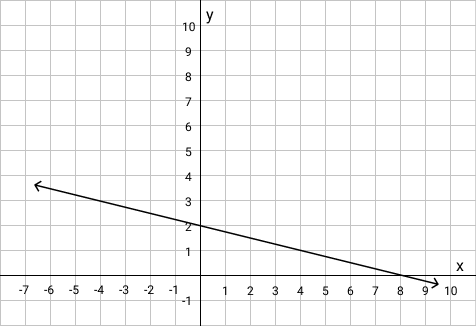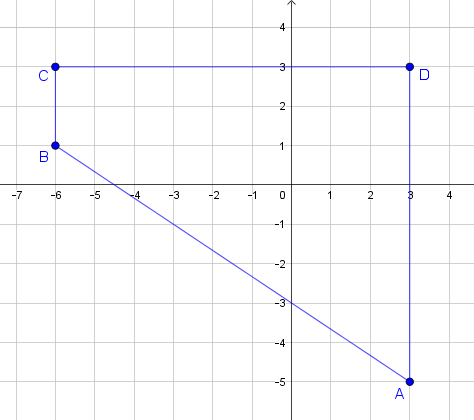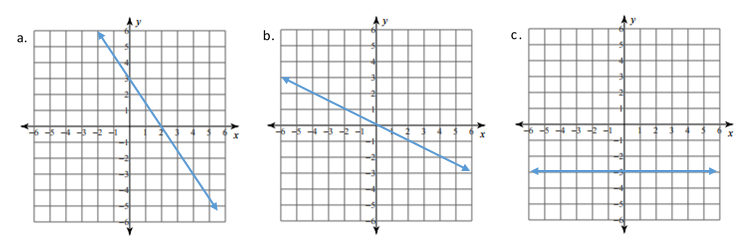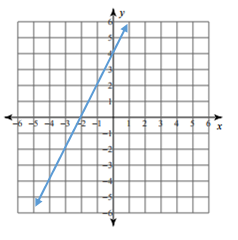# Linear Relationships

## Objective

Write linear equations from graphs in the coordinate plane.

## Common Core Standards

### Core Standards

?

• 8.EE.B.6 — Use similar triangles to explain why the slope m is the same between any two distinct points on a non-vertical line in the coordinate plane; derive the equation y = mx for a line through the origin and the equation y = mx + b for a line intercepting the vertical axis at b.

• 8.F.B.4 — Construct a function to model a linear relationship between two quantities. Determine the rate of change and initial value of the function from a description of a relationship or from two (x, y) values, including reading these from a table or from a graph. Interpret the rate of change and initial value of a linear function in terms of the situation it models, and in terms of its graph or a table of values.

## Criteria for Success

?

1. Identify the slope and $y$-intercept of a line graphed in a coordinate plane.
2. Given a graph of a line, write a linear equation in slope-intercept form.
3. Match graphs of equations and equations written in slope-intercept form.

## Tips for Teachers

?

• Anchor Problem #3 is an optional matching game activity that requires preparation in advance of the lesson.
• The following materials will be needed for this lesson: Matching Game.

#### Fishtank Plus

• Problem Set
• Student Handout Editor
• Vocabulary Package

## Anchor Problems

?

### Problem 1

What is the equation of the line represented in the graph below?### Problem 2

Four lines were used to define the edges of the trapezoid shown below.Write an equation for each line described below:

1. Line that passes through points A and B
2. Line that passes through points B and C
3. Line that passes through points C and D
4. Line that passes through points D and A

### Problem 3

Optional: Play the memory Matching Game. Note that this requires some advance preparation.

Directions for students:

1. Find a partner and clear off your desks.
2. Separate the cards into a pile with equations and a pile with graphs.
3. Turn the equation cards over and organize them into rows/columns on one desk.
4. Turn the graph cards over and organize them into rows/columns on another desk.
5. On the first person’s turn, turn over an equation card and a graph card. If the cards match, then the player gets to keep the cards. If they do not match, turn the cards over in the same spot. The turn is over.
1. NOTE: If the player believes she or he has a match, then the player must prove that there is a match. The other player has the option to disagree and explain why. If the other player is right (and the first player was mistaken), then the other player gets to keep the card and then take a turn.
6. Repeat step 5 for the second player’s turn.
7. The game is over when all cards have been matched. The person with the most number of pairs wins the round. Another round may be played.

## Problem Set

?The following resources include problems and activities aligned to the objective of the lesson that can be used to create your own problem set.

• Include spiraled problems of finding slope from two coordinate points.

?

### Problem 1

Write the slope-intercept form of the equation for each line below.### Problem 2

Marty writes the equation ${y=4x+2}$ to represent the line shown in the graph below. Do you agree with the equation that Marty wrote for the line? Explain why or why not.?